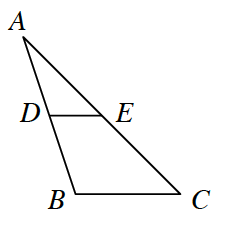### Home > GC > Chapter 9 > Lesson 9.1.3 > Problem9-29

9-29.

In the diagram at right, $\overline{DE}$ is a midsegment of $ΔABC$. If the area of $ΔABC$ is $96$ square units, what is the area of $ΔADE$? Explain how you know.$ΔADE\simΔABC$

What is the linear scale factor?
What is the ratio of the areas?

$24$ square units.